﻿
Reach Us+44-7482-875032
Unsteady Natural Convection Flow through a Vertical Channel: Due to the Combined Effects of Variable Viscosity and Thermal Radiation

# Journal of Applied & Computational MathematicsOpen Access

All submissions of the EM system will be redirected to Online Manuscript Submission System. Authors are requested to submit articles directly to Online Manuscript Submission System of respective journal.

# Unsteady Natural Convection Flow through a Vertical Channel: Due to the Combined Effects of Variable Viscosity and Thermal Radiation

Ajibade AO* and Bichi YA
Department of Mathematics, Ahmadu Bello University, Zaria, Nigeria
*Corresponding Author: Ajibade AO, Department of Mathematics, Ahmadu Bello University, Zaria, Nigeria, Tel: +2348068843483, Email: [email protected]

Received Date: May 28, 2018 / Accepted Date: Jul 10, 2018 / Published Date: Jul 18, 2018

Keywords: Natural convection; Variable viscosity; Thermal radiation; ADM

#### Nomenclature and Greek symbols

 Symbols Interpretation Unit y' Dimensional length m y Dimensionless length t Time s g Acceleration due to gravity ms-2 k Thermal conductivity W/mK T Dimensional temperature k h Dimensional width of the channel m H Dimensional channel width m Tw Wall temperature K T0 Ambient temperature K u,v Dimensional velocity ms-1 f Dimensionless velocity v Kinematic viscosity of the fluid m2s-1 α Thermal diffusivity m2 s-1 δ Absorption coefficient β Volumetric expansion coefficient K-1 μ Variable fluid viscosity kgm-1s-1 μ0 Dynamic viscosity of the fluid kgm-1 s-1 qr Radiative heat flux Wm-2 θ Temperature difference parameter K θ Dimensionless temperature σ Stefan-Boltzman constant α Viscosity variation parameter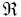Set of real numbers Nu0 Nusselt number at the plate y-0 Nu1 Nusselt number at the plate y=1 τ0 Skin friction at the plate y=0 τ1 Skin friction at the plate . y=1

#### Introduction

The study of fluid flow with variable viscosity has become of principal interest in many scientific and engineering applications, such as crude oil extraction, food processing, coating and polymer processing industries. In industrial systems, fluid can be subjected to extreme conditions such as high temperature, pressure and shear rate. External heating and high shear rate can lead to a high temperature being generated in the fluid; this leads to a significant effect on the fluid properties [1,2]. It is well known that the most sensitive fluid property to temperature rise is the viscosity and this is reported in the works of Johns and Narayanan , Hashemabadi et al. , Becker and Kingsley . For many liquids, among them water, petroleum oils, glycerin, glycols, silicone fluids and some molten salts, the percent variation of absolute viscosity with temperature is much more than that of the other properties. For instance, when the temperature increases from 10°C (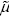= 0.0133g / cms) to 50°C (= 0.00548g / cms) the viscosity of water decreases by 240% Carey and Mollendorf . Fluids used in industries, such as polymer fluids have viscosities that vary rapidly with temperature and this may give rise to strong feedback effects . The usual assumption of constant viscosity property of fluid evaluated at some reference temperature is not enough to depict the true picture in the flow characteristics in boundary layer flows. Garey et al. , Mehta and Sood  lamented that when varying viscosity property of fluid is included, the flow characteristics change substantially compared to the constant case. Kafousius et al. , Kafousius and Williams  investigated the effect of temperature-dependent viscosity on mixed convection past a vertical flat plate in the region near a leading edge and concluded that when the viscosity of the fluid is sensitive to temperature variation, the effect of temperature-dependent viscosity has to be taken into consideration; otherwise, considerable errors may occur in the characteristics of the heat transfer process. Entropy generation is a measure of destruction of the available work done by a system. When it occurs, the consequent effect is the emission of heat in the form of electromagnetic rays termed as thermal radiation. In order to minimize entropy generation, several studies were carried out to this effect; for instance, Ajibade et al.  studied entropy generation under the effect of suction/injection and concluded that entropy generation increases with suction on one plate and it decreases on the other plate with injection. Makinde  and Ibanez et al.  lamented that when entropy generation takes place, the quality of energy of a system decreases.

Radiation effects on the free convection flow are important in the context of space technology and processes involving high temperature. In view thereof, Makinde and Ogulu  analyzed the effect of thermal radiation on the heat and mass transfer flow of a variable viscosity fluid past a vertical porous plate permeated by a transverse magnetic field where they concluded that an increase in the positive value of viscosity variation parameter decreases the fluid viscosity and consequently leads to an increase in the velocity within the boundary layer. Other similar researches can be seen in Hossain et al. , Seddeek and Salem , Elbasbeshy and Bazid , Costa and Macedonio . The expression for radiative heat flux term which is widely used in the study of heat transfer process in boundary layer flows was given by Sparrow and Cess. . Despite its shortcomings of being valid for intensive absorption, that is, for optically thick boundary layer flows; several researchers have used it with success. This can be seen in Makinde et al. , Makinde and Ogulu , and Makinde and Ibrahim . The latter researchers used a linearised form of temperature in the expression for radiative heat flux during their studies. However, this was condemned by Pantokratoras and Magyari  on the basis that; the linearized form of temperature in most boundary layer flows with thermal radiation does not portrays a real characteristics in heat emission or conduction, as such it is uncalled for any publications neither does it require any additional research efforts. They therefore proposed alternative approach in where temperature in the radiative heat flux is evaluated using normal differentiation.

In the field of science and engineering, there are few phenomena that occur linearly while most occur non-linearly. Non-linear equations are difficult to handle and some can be solved using analytical methods, semi-analytical methods or traditional numerical methods such as Homotopy analysis method (HAM), variational iteration method (VIM), Runge-Kutta method, and finite difference method. The other well-known method is the ADM proposed by Adomian . Numerous researchers have used this technique in their studies, this can be viewed , Adesanya et al. , Adesanya and Makinde , Adesanya and Cbadeyan , Venkatarangan and Rajalakshmi  and Makinde et al.  a few to mention among others.

In the present article, we consider unsteady natural convection flow due to the combined effects of variable viscosity and thermal radiation which will be analysed Cherruault  by non-linear Rosseland diffusion approximation. Similarly, the fluid dynamic viscosity is considered to vary linearly with the fluid temperature following .

#### Mathematical Formulation

The physical problem under consideration consists of a vertical channel formed by two vertical parallel plates; stationed h distance apart. The channel is filled with viscous incompressible fluid in the presence of thermal radiation with all the fluid physical properties assumed constant except for its viscosity which is temperature dependent. Similarly, the fluid is considered optically thick where the radioactive heat flux of Roseland diffusion approximation can be utilized. The x' -axis is taken along the channel in the vertically upwards direction, being the direction of the flow while the x' -axis is taken normal to it. In addition, the effects of radiative heat flux in the x'-direction is considered negligible compared with that in the y' -direction. At time y=0, both the fluid and the plates are assumed to be at rest with constant temperature T0 . At time t>0, the temperature of the plate kept at y=0 rise to Tw while the other plate at y=h is fixed and maintained at temperature T0. The coordinates system and the flow configuration are shown in Figure 1 below:

Under these assumptions and on using Bouss in eqn. approximation, the appropriate governing equations are: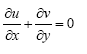(1)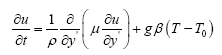(2)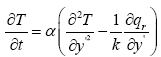(3)

where u, v are the velocity components in the x' and y' directions respectively, ρ is the density of the ambient fluid g; the acceleration due to gravity, β is the coefficient of thermal expansion k; the coefficient of thermal conductivity, T ; the temperature of the fluid, y' is the ambient fluid temperature and qr is the radiative heat flux in the y' direction.

Following Carey and Mollendorf , the fluid dynamic viscosity is of the form: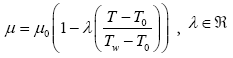(4)

and the radiative heat flux term (qr) as given by Sparrow and Cess  is: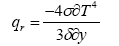(5)

with the boundary conditions for the velocity and temperature fields as:

u=0,T=Tw at y' = 0

u=0,T=T0 at y' = h (6)

The following dimensionless quantities are introduced: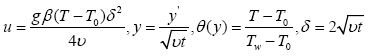(7)

using eqns. (4), (6) and (7) in eqn. (2) we have:(8)

Similarly, using eqns. (5-7) in eqn. (3) give: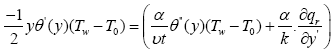(9)

The term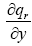; containing the radioactive heat flux term (qr) in the right hand side of eqn. (9) is simplified following Pantokratoras and Magyari  as follows: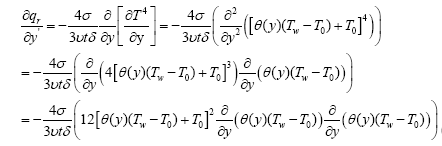(10)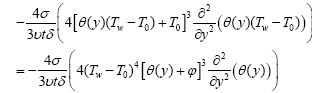Substituting eqn. (10) in eqn. (9) gives: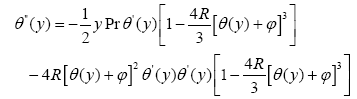(11)

Furthermore, using eqn. (6) in eqn. (4), the boundary conditions are now:

f(0)=0,θ(0)=1.f(H)=θ(H)=0 (12)

Where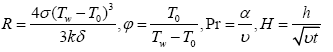(13)

Pr represents Prandt l number which is inversely proportional to the thermal diffusivity α of the working fluid and θ is temperature difference parameter where R is the radiation parameter. The meanings of the other physical quantities involved are given in the nomenclature.

Description of the ADM

Consider the inhomogeneous nonlinear differential equation in Adomian’s operator-theoretic form

Lu+Su+Nu=g (14)

Where u is unknown function or system output, which is to be determined by a recursive relation, L is the invertible linear operator which is the highest order derivative S, is the remainder of the linear operator whose order is less than L, Nu represents the nonlinear terms and g is the source term or system input.

operating L−1 to both sides of and using the given initial boundary conditions, we obtain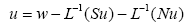(15)

Where w represents the term arising from integrating the source term g and the auxiliary conditions

The standard ADM defines the solution by the decomposition series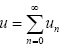(16)

and the nonlinear term comprises the series of the Adomian polynomials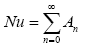(17)

where the An are the Adomian polynomials generated from the relation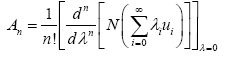(18)

such that the Adomian polynomials are evaluated as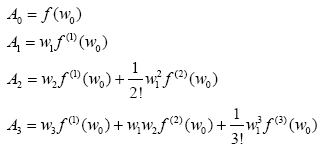(19)

The solution components u0,u1,u2,……are determined recursively as follows

u0=w (20)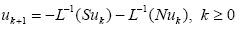(21)

Where w0 is referred to as the zeroth-order component.

Adomian decomposition solution of the problem

Eqns. (10) and (11) under the boundary conditions (12) are solved as follows:

Denote by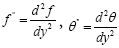and let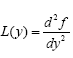, so that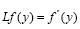and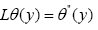(22)

then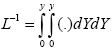(23)

eqn. (10) and eqn. (11) can now be written as: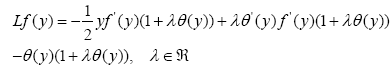(24)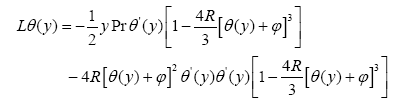(25)

Operating L-1 on both sides of eqns. (24) and (25), we obtain: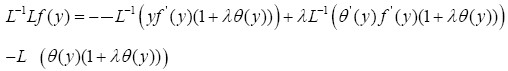(26)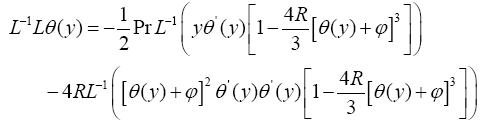(27)

By ADM: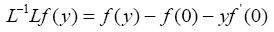(28)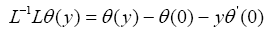(29)

Using eqns. (10), (28) and (29) in eqns. (26) and (27), respectively we have: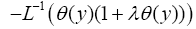(30)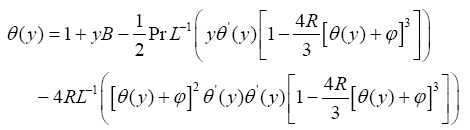(31)

According to the standard ADM,f(y) and θ(y) may be expressed as: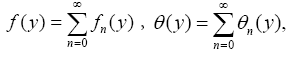(32)

Putting eqn. (32) in eqns. (30) and (31) respectively, we have: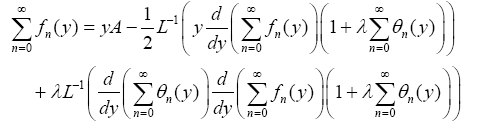(33)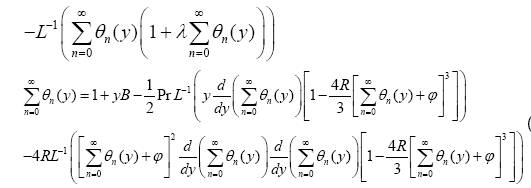(34)

Setting f0(y)=Ay and θ0(y)=1+By then fj+1(y) and θj+1(y) for j≥0 are determined using the recursive relations: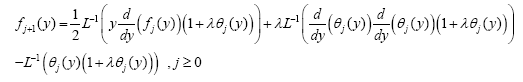(35)

and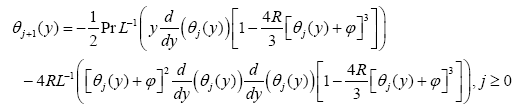(36)

Convergence analysis

Convergence of ADM solution has been given in Adomian  and Charruault . Despite this, to verify the convergence of our ADM solution in the present problem, we deploy the method of ratio test for convergence. Using computer simulation, the following ADM series of eqn. (35 and 36) at y=0.5, R=0.1,θ=0.1,λ=0.1,Pr=0.71 are obtained as follows:

θ0=0.4729720015, θ2=-0.00008134291586, θ3=0.0000008523379614 , θ4=-4.4144946266×10-10, f0=0.06376280558, f1=-0.004159525801, f2=0.0 0009144558899, f3=-0.00000159539894, f4=2.202779961×10-8.

On the application of ratio test, it can be seen that; all the terms in eqn. (37) satisfied the ratio test formula for convergence i.e.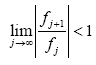. Hence, the ADM solution in the present problem converges.

Termination criterion

For an infinite series solution to be meaningful, the solution needs to be terminated at a point such that any additional term contributes insignificantly to the final solution. Our solution is thus truncated whenever |fj|<δ and |θj|<ξ. For the present problem, we have chosen δ=1.6*10-7and ξ=8.5*10-7. Based on this assumption, the approximate solution is truncated after the 3rd term. Due to the cumbersome of the computed ADM series solution obtained via computer coded simulation; only numerical and graphical results are presented for the purpose of discussion.

Nusselt numbers on the channel walls are obtained respectively via: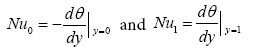(38)

and the skin friction on the walls are calculated using: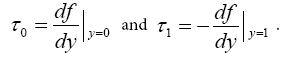(39)

Validation of the result

Eqns. (8) and (11) representing the flow governing equations are highly non-linear differential equations. In order to validate our result, we compare the ADM solution of these equations with that achieved via series solution of the same problem in the absence of thermal radiation and viscosity variation parameter, that is setting λ=0 in eqn. (8) and R=0 in eqn. (11) we obtain the followings differential equations: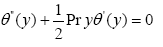(40)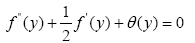(41)

Applying series method of solution to solve eqn. (40) is as follows: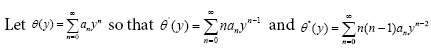(42)

Substituting eqn. (42) in eqn. (40) together with the boundary conditions (12) in eqn. (12) and simplifying, we obtained the following series solution: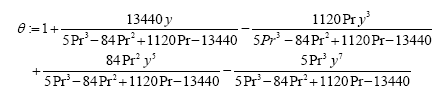(43)

Similarly; applying series method to solve eqn. (41) is as follows:

Let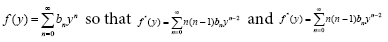(44)

Substituting eqn. (44) together with the boundary conditions (12) and eqn. (43) in eqn. (41) we obtained the following series solution: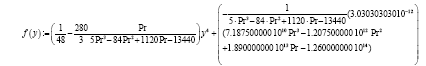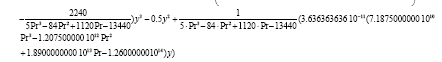(45)

#### Results and Discussions

Using computer algebra software package, eqns. (35) and (36) are simulated and the results are presented graphically as velocity and temperature profiles in order to examine the effects of the physical parameters involved in the problem. For the purpose of discussion, the value of Pr has been chosen as 0.015, 0.71, 1.0 and 4.0 which correspond to important fluids; mercury, air, electrolyte solution and refrigerant respectively. The values of radiation parameter (R), temperature difference parameter θ and viscosity parameter λ are chosen between 0.1 and 0.4

Figure 2 demonstrates the effects of varying Prandtl number (Pr) on the fluid temperature. It is noticed that the temperature of the fluid within the channel decreases as Pr increases. This due to the fact that, fluid with higher Pr possesses lower thermal conductivity and as such heat diffuse slower than in fluid with lower Pr. The consequent effect of the decrease in the heat generation within the channel has gone further to affect the fluid velocity; this can be seen in Figure 3. This figure shows that, when Pr increases, it strengthen cohesive force between the fluid molecules and as such fluid with higher Pr flow slowly than fluid with lower Pr. The effect of thermal radiation (R) is observed in Figure 4, this figure shows that the fluid velocity increases with an increase in R. This is owing to the fact that; when R increases, the thermal conductivity of the fluid within the channel is also increased. The transitive effect of this is reflected in Figure 5, where the fluid velocity is also seen to increase with increasing R. This is owing to the fact that, the buoyancy force of the fluid is increased there by making flow fast. Similarly, the effect of temperature difference parameter (ɸ) is illustrated in Figure 6 where the fluid temperature is observed to increases with increase in ɸ. Thus, when (Tw−T0) is decreased, the consequent result is a corresponding increase in ɸ. In the same vein, Figure 7 shows the velocity profile under the effect of ɸ. It is viewed from the figure that, the velocity increases with an increase in ɸ. Increasing the value of ɸ loosens the cohesive force between the fluid molecules and this resulted into speedy flow of the fluid. Finally, the effect of viscosity parameter (λ) is revealed in Figure 8. It portrays that, the fluid velocity increases with increase in λ. The increase in velocity is caused by the decrease in viscosity of the fluid when increases.

Table 1 shows comparison between ADM and series solution of the present problem when R=0 and λ=0. The table displayed that, the solution of the problem under study by ADM coincides with that of the same problem obtained using series solution method all in the absence of thermal radiation and viscosity variation parameter.

y ADM Solution
Pr=0.71, R=0, θ(y)
Series Solution
Pr=0.71, R=0
θ (y)
ADM Solution
Pr=0.71, R=0, λ =
0 f(y)
Series Solution
Pr=0.71, R=0, λ=0
f(y)
0.1 0.8941137051    0.8941137052 0.0276449020 0.02759155975
0.2 0.7886025293    0.7886025294 0.0462402538 0.04611689205
0.3 0.6838376099    0.6838376100 0.0568085498 0.05656709524
0.4 0.5801821910    0.5801821912 0.0604331342 0.05999580516
0.5 0.4779878556    0.4779878559 0.0582388612 0.0575191945
0.6 0.3775909696    0.3775909699 0.0513730368 0.0503159738
0.7 0.2793094114    0.2793094117 0.0409867369 0.0396273906
0.8 0.1834396557    0.1834396559 0.0282165962 0.0267572296
0.9 0.0902542855    0.0902542856 0.0141671603 0.0140718130
1.0 6.4000x10-10    5.59448 × 10-17   0.000106104 3.00 × 10-10

Table 1:  Computations showing comparison between ADM and series solution.

The effects of Prandtl numbers on the Nusselt number are shown in Table 2. It is observed that; the Nusselt number on the channel wall stationed at y=0 increases with increase in Pr while it decreases with increase in Pr on the wall kept at y=1. Furthermore, the Nusselt number on the wall fixed at y=0 is observed to decrease with increase in R whereas it is found to increase with increase in R on the wall at y=1; all at maintained value of Pr. Once more, the Nusselt number on the wall at y=0 is noticed to decrease with increase in θ but it is seen to increase with increase in θ on the wall at y=1; all at fixed value of Pr.

Pr R=0.1,   θ=0.21 R=0.2, θ=0.21 R=0.2,0,θ=0.5
Nu0 Nu1 Nu0 Nu1 Nu0 Nu1
0.015 0.883279 1.06001  0.825257 1.107800 0.824158 1.171530
0.71 0.924742 0.956932 0.856568 1.017510 0.850279 1.097740
1.00 1.004730 0.86902 0.872138 0.986143 0.837041 0.921653
4.00 1.197150 0.278266 1.054080 0.448140 0.984510 0.647312

Table 2: Numerical values for the rate of heat transfer on the channel walls.

The Table 3 above shows the effects of viscosity variation parameter (λ) on the skin friction. It can be viewed that; the skin friction on the channel walls decreases with increase in λ. Moreover, the skin friction on the walls is found to increase with increase in R and θ; all for a fixed value of λ.

R=0.1,θ =0.21,Pr=0.71 R=0.2,θ =0.21,Pr=0.71 R=0.2,θ =0.5 , Pr=0.71
τ0 τ1 τ 0 τ1 τ 0 τ
0.1 0.671850 0.327637  0.687608 0.338249 0.699419 0.348278
0.2 0.636111 0.275282 0.655659 0.284512 0.670454 0.293241
0.4 0.541832 0.153239 0.570121 0.161570 0.591764 0.167642
0.6 0.416437 0.013986 0.455034 0.017246 0.484687 0.020591

Table 3: Numerical values of skin friction on the walls.

#### Conclusion

This article studied unsteady natural convection flow with thermal radiation in a vertical channel by invoking non-linear Rosseland diffusion approximation in the energy equation. The model representing the present physical situation is highly non linear due to the presence of thermal radiation effect. A semi-analytical method (ADM) together with computer algebra package for simulation has been deployed for the solution of the flow equations. The impacts of the essential controlling physical parameters involved in the problem are both graphically and computationally examined. Furthermore; comparison between the ADM and series solutions of the studied problem; in the absence of thermal radiation has been carried out in Table 1 to validate our result. Nusselt numbers and skin frictions on the bounded channel walls has also been presented in Tables 2 and 3 and discussed. The following results were deduced from the study:

i. The fluid velocity and its temperature within the channel were found to decrease with increase in Prandtl numbers.

ii. The temperature of the fluid and its velocity in the flow model were both seen to decrease with increase in thermal radiation and temperature difference parameter respectively.

iii. Increase in viscosity variation parameter was noticed to results into corresponding increase in the fluid velocity within the channel.

iv. Numerical comparison between the ADM solution and the series solution of the studied problem when both thermal radiation and viscosity variation parameter were relaxed has been conducted and presented in Table 1. The result shows an excellent agreement between the two methods.

v. It is worthy to mention here that; when viscosity of any working fluid is sensitive to temperature change, the effects of variable viscosity has to be taken into account otherwise considerable errors may occur.

vi. This study is hoped to serve as a compliment to previous ones and also be an avenue for further researches.

#### References

Citation: Ajibade AO, Bichi YA (2018) Unsteady Natural Convection Flow through a Vertical Channel: Due to the Combined Effects of Variable Viscosity and Thermal Radiation. J Appl Computat Math 7: 403. DOI: 10.4172/2168-9679.1000403

Copyright: © 2018 Ajibade AO, et al. This is an open-access article distributed under the terms of the Creative Commons Attribution License, which permits unrestricted use, distribution, and reproduction in any medium, provided the original author and source are credited.

Select your language of interest to view the total content in your interested language

##### Recommended Journals
Viewmore
###### Article Usage
• Total views: 816
• [From(publication date): 0-2018 - Aug 21, 2019]
• Breakdown by view type
• HTML page views: 734
• PDF downloads: 82

## Post your commentCan't read the image? click here to refresh
###### Peer Reviewed Journals

Make the best use of Scientific Research and information from our 700 + peer reviewed, Open Access Journals

International Conferences 2019-20

Meet Inspiring Speakers and Experts at our 3000+ Global Annual Meetings

Top